### Home > MC2 > Chapter 6 > Lesson 6.2.4 > Problem6-110

6-110.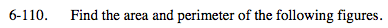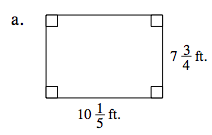Remember, the perimeter is the sum of all sides while the area is the value of the space within the perimeter.

Area = (base) (height)
Perimeter of a rectangle = 2 (base) + 2 (height)

$A=79\frac{1}{20}\text{f}t^{2}$

$P=35\frac{9}{10}\text{ft}$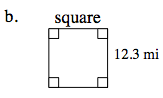Remember, a square has 4 sides of equal lengths.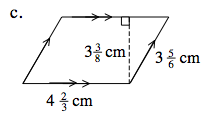The area of a parallelogram is found by multiplying the base by the height. The perimeter is the sum of the 4 sides.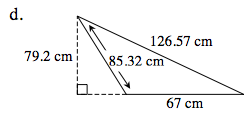The area of a triangle is found by multiplying the base by the height and dividing that product by 2.

A = 2653.2 cm2
P = 278.89 cm